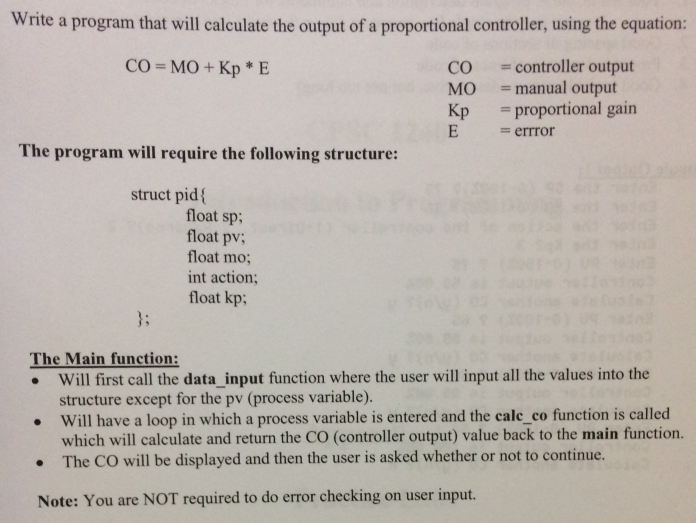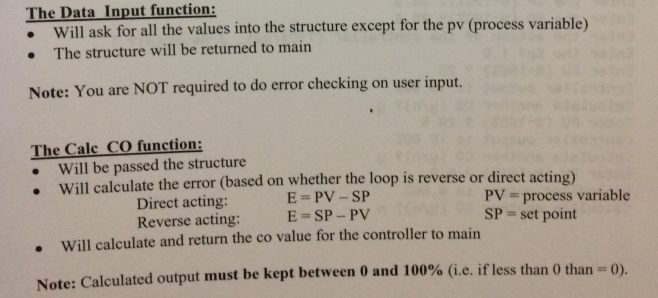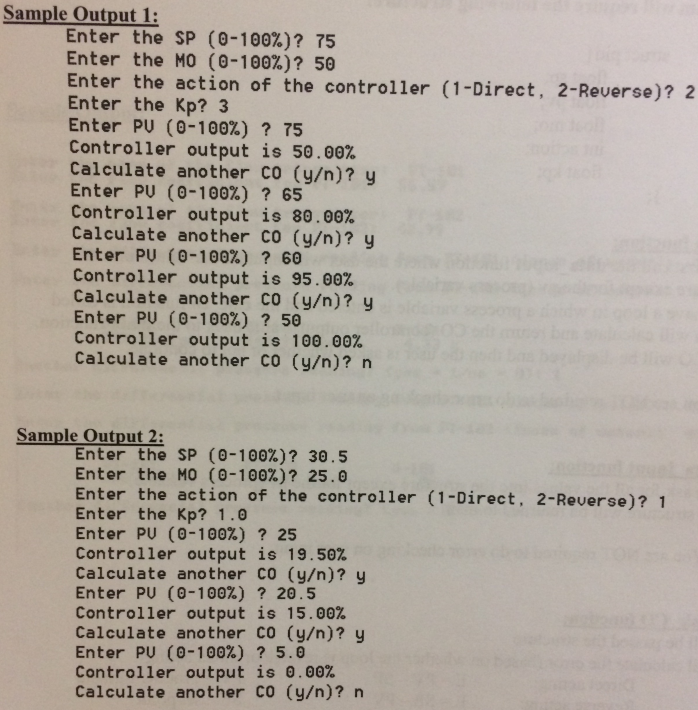# (Solved) : Write Program Calculate Output Proportional Controller Using Equation Co Mo Kp E Co Contro Q42761697 . . .

write the detailed program with C++VisualStudioWrite a program that will calculate the output of a proportional controller, using the equation: CO = MO+Kp * E CO = controller output = manual output = proportional gain = errror The program will require the following structure: struct pid float sp; float pv; float mo; int action; float kp; The Main function: • Will first call the data input function where the user will input all the values into the structure except for the pv (process variable). • Will have a loop in which a process variable is entered and the cale co function is called which will calculate and return the CO (controller output) value back to the main function. • The CO will be displayed and then the user is asked whether or not to continue. Note: You are NOT required to do error checking on user input. The Data Input function: • Will ask for all the values into the structure except for the pv (process variable) • The structure will be returned to main Note: You are NOT required to do error checking on user input. The Calc CO function: Will be passed the structure . Will calculate the error (based on whether the loop is reverse or direct acting) Direct acting: E-PV-SP PV = process variable Reverse acting: E=SP- PV SP = set point . Will calculate and return the co value for the controller to main Note: Calculated output must be kept between 0 and 100% (i.e. if less than 0 than -0). Sample Output 1: Enter the SP (0-100%)? 75 Enter the MO (0-100%)? 50 Enter the action of the controller (1-Direct, 2-Reverse)? 2 Enter the Kp? 3 Enter PU (0-100%) ? 75 Controller output is 50.00% Calculate another CO (y/n)? y Enter PU (0-100%) ? 65 Controller output is 80.00% Calculate another co (y/n)? y Enter PU (0-100%) ? 60 Controller output is 95.00% Calculate another Co (y/n)? y Enter PU (0-100%) ? 50 Controller output is 100.00% Calculate another co (y/n)? n Sample Output 2: Enter the SP (0-100%)? 30.5 Enter the MO (0-100%)? 25.0 Enter the action of the controller (1-Direct. 2-Reverse)? 1 Enter the Kp? 1.0 Enter PU (0-100%) ? 25 Controller output is 19.50% Calculate another CO (y/n)? y Enter PU (0-100%) ? 20.5 Controller output is 15.00% Calculate another co (y/n)? y Enter PU (0-100%) ? 5.0 Controller output is 0.00% Calculate another co (y/n)? n Show transcribed image text Write a program that will calculate the output of a proportional controller, using the equation: CO = MO+Kp * E CO = controller output = manual output = proportional gain = errror The program will require the following structure: struct pid float sp; float pv; float mo; int action; float kp; The Main function: • Will first call the data input function where the user will input all the values into the structure except for the pv (process variable). • Will have a loop in which a process variable is entered and the cale co function is called which will calculate and return the CO (controller output) value back to the main function. • The CO will be displayed and then the user is asked whether or not to continue. Note: You are NOT required to do error checking on user input.
The Data Input function: • Will ask for all the values into the structure except for the pv (process variable) • The structure will be returned to main Note: You are NOT required to do error checking on user input. The Calc CO function: Will be passed the structure . Will calculate the error (based on whether the loop is reverse or direct acting) Direct acting: E-PV-SP PV = process variable Reverse acting: E=SP- PV SP = set point . Will calculate and return the co value for the controller to main Note: Calculated output must be kept between 0 and 100% (i.e. if less than 0 than -0).
Sample Output 1: Enter the SP (0-100%)? 75 Enter the MO (0-100%)? 50 Enter the action of the controller (1-Direct, 2-Reverse)? 2 Enter the Kp? 3 Enter PU (0-100%) ? 75 Controller output is 50.00% Calculate another CO (y/n)? y Enter PU (0-100%) ? 65 Controller output is 80.00% Calculate another co (y/n)? y Enter PU (0-100%) ? 60 Controller output is 95.00% Calculate another Co (y/n)? y Enter PU (0-100%) ? 50 Controller output is 100.00% Calculate another co (y/n)? n Sample Output 2: Enter the SP (0-100%)? 30.5 Enter the MO (0-100%)? 25.0 Enter the action of the controller (1-Direct. 2-Reverse)? 1 Enter the Kp? 1.0 Enter PU (0-100%) ? 25 Controller output is 19.50% Calculate another CO (y/n)? y Enter PU (0-100%) ? 20.5 Controller output is 15.00% Calculate another co (y/n)? y Enter PU (0-100%) ? 5.0 Controller output is 0.00% Calculate another co (y/n)? n

Answer to Write a program that will calculate the output of a proportional controller, using the equation: CO = MO+Kp * E CO = con…

We are the best freelance writing portal. Looking for online writing, editing or proofreading jobs? We have plenty of writing assignments to handle.